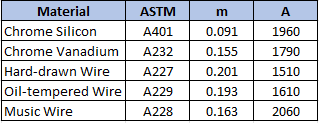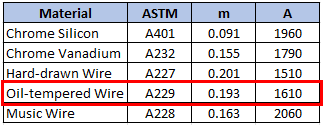## Spring Tensile Strength

An oil-tempered wire with a diameter of 10 mm will be used as a spring material in an assembly. Calculate the minimum tensile strength of the wire.Hint
For spring materials, the minimum tensile strength for common spring steels can be determined from:
$$S_{ut}=\frac{A}{d^{m}}$$$where $$S_{ut}$$ is the tensile strength in MPa, and $$d$$ is the wire diameter in millimeters. Hint 2 $$A$$ and $$m$$ can be found in the below table:For spring materials, the minimum tensile strength for common spring steels can be determined from: $$S_{ut}=\frac{A}{d^{m}}$$$
where $$S_{ut}$$ is the tensile strength in MPa, $$d$$ is the wire diameter in millimeters, and $$A$$ and $$m$$ can be found in the below table:Since the problem is asking for the minimum tensile strength of an oil-tempered wire:
$$S_{ut}=\frac{1610}{(10mm)^{0.193}}=\frac{1610}{1.5596}=1,032\: MPa$$\$
1,032 MPa